# Formulas for surface and volume

## The formulas for surface and volume

Formulas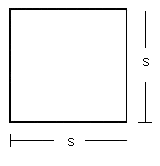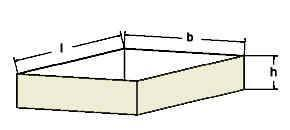The area of the square or rectangle = side x side.

The volume of a box = height x length x width.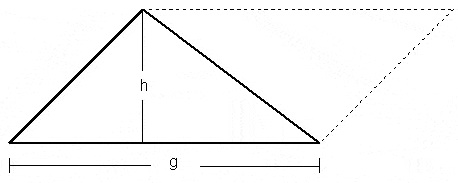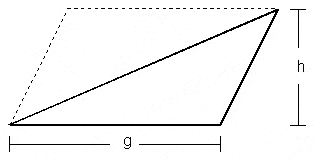The Area of a triangle = 1/2 x base (g) x height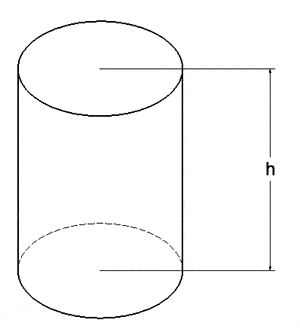PI = ca. 3,14

Circle’s circumference = Diameter x PI